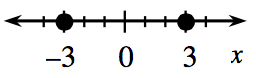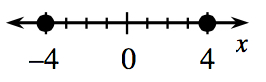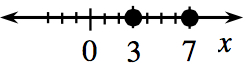### Home > INT2 > Chapter 8 > Lesson 8.2.2 > Problem8-45

8-45.

One way to look at absolute value is to think of the absolute value of a number as its distance from zero on a number line. For example, $\left| 6 \right| = 6$ and $| −6 | = 6$
and $| −6 | = 6$ $= 6$ because both $6$ and $-6$ are exactly $6$ units from zero on a number line. You can, therefore, think of the equation such as $|x|= 5$ as the question, “What numbers are exactly five units from zero on the number line?” Thus, the solutions are $5$ and $-5$. Draw a number line to show the solutions to the following equations.

1. $|x|= 3$1. $|x|= 0$

1. $|x|+ 2 = 6$1. $3|x|= 15$

1. $| x - 5 |= 2$1. $| x + 3 |= 7$